# Right triangle + arctangent - math problems

#### Number of problems found: 32

• Ratio iso triangleThe ratio of the sides of an isosceles triangle is 7:6:7 Find the base angle to the nearest answer correct to 3 significant figure.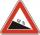Road sign informs the gradient is 10.9%. Calculate the angle which average decreases.
• BevelI have bevel in the ratio 1:6. What is the angle and how do I calculate it?
• ClimbRoad has climbing 1:27. How big is a angle corresponds to this climbing?On a straight stretch of road is marked 12 percent drop. What angle makes the direction of the road with the horizontal plane?
• Height differenceWhat height difference overcome if we pass road 1 km long with a pitch21 per mille?
• Trapezoid MOThe rectangular trapezoid ABCD with the right angle at point B, |AC| = 12, |CD| = 8, diagonals are perpendicular to each other. Calculate the perimeter and area of ​​the trapezoid.Average climb of the road is given by ratio 1:15. By what angle road average climb?
• RailwayRailway line had on 5.8 km segment climb 9 permille. How many meters track ascent?
• Hole's angles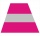I am trying to find an angle. The top of the hole is .625” and the bottom of the hole is .532”. The hole depth is .250” what is the angle of the hole (and what is the formula)?
• Cube - anglesCalculate angle between the wall diagonal and cube base. Calculate the angle between the cube body diagonal and cube base.
• StairwayWhat angle rising stairway if step height in 20 cm and width 26 cm?
• V-belt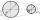Calculate a length of the V-belt when the diameter of the pulleys is: D1 = 600 mm D2 = 120 mm d = 480 mm
• Church tower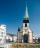Archdeacon church in Usti nad Labem has diverted tower by 186 cm. The tower is 65 m high. Calculate the angle by which the tower is tilted. Result write in degree's minutes.
• Traffic signThere is a traffic sign for climbing on the road with an angle of 7%. Calculate at what angle the road rises (falls).Between cities A and B is route 13 km long of stúpanie average 7‰. Calculate the height difference of cities A and B.
• ConeThe rotating cone volume is 9.42 cm3, with a height 10 cm. What angle is between the side of the cone and its base?
• Angle of two linesThere is a regular quadrangular pyramid ABCDV; | AB | = 4 cm; height v = 6 cm. Determine the angles of lines AD and BV.
• V-belt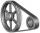Calculate the length of the belt on pulleys with diameters of 105 mm and 393 mm at shaft distance 697 mm.
• PyramidPyramid has a base a = 3cm and height in v = 15 cm. a) calculate angle between plane ABV and base plane b) calculate angle between opposite side edges.

Do you have an interesting mathematical word problem that you can't solve it? Submit a math problem, and we can try to solve it.

We will send a solution to your e-mail address. Solved examples are also published here. Please enter the e-mail correctly and check whether you don't have a full mailbox.

Please do not submit problems from current active competitions such as Mathematical Olympiad, correspondence seminars etc...

See also our right triangle calculator. Right triangle Problems. Arctangent - math word problems.